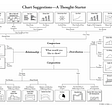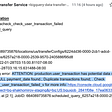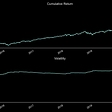# Pricing Interest Rate Options using Python

Interest Rates are a key component in every economy. They are linked to almost every economic activity happening in the market be it lending/borrowing of funds, pricing of financial products, trading, asset-liability management for banks etc. Just as interest rates are important in the cash market, they play an equal or albeit more significant in the derivatives market.

Derivatives as a product type are widely used for various applications in finance be it trading, risk management, hedging etc. Derivatives particularly those in the OTC market can be structured in any way and can have any underlying asset as long as there is a counterparty to trade this derivative with.

Derivatives that have interest rates as an underlying are called as Interest Rate derivatives. These may be Futures, Forward Rate Agreements (FRAs), Swaps, Options etc. In this post we will discuss about Interest Rate options. We will specifically talk about Interest Rate Caps. The article will explain the structure of Caps, their pricing methodology and finally we will discuss the Python implementation of the pricing model for Interest Rate Caps.

What are Caps?

Caps are a type of an interest rate option. Imagine Cap to be a bundle of options. For instance, assume a European Cap with maturity 1 year, and has 4 resets (i.e. it’s a bundle of 4 options). These individual options are called as caplets. Mathematically, we can represent this as:

Each of the four caplets are individual options in themselves. Thus, each caplet can be priced separately. The final MTM / pricing of a Cap is an addition of the four individual caplets. This is the basic structure of a Cap in a nutshell

Pricing Methodology

The market generally uses a Black’s model for pricing of Caps. Thus, the Black’s model will be used to price the individual caplets.

1. Assume a 1-year Cap as we mentioned above, we will assume a quarterly frequency on this Cap i.e. there are four caplets, lets represent then as c1, c2, c3 and c4

2. Let the underlying interest rate be USD LIBOR

Let’s say, the Cap contract has commenced and we are 30 days into the contract. At each of the reset dates, LIBOR rate will be observed and compared against the Cap Strike rate. If the LIBOR rate > Cap strike, then the respective Caplet gives a positive payoff. Caps are generally defined so that the initial LIBOR rate, even if it is greater than the cap rate, does not lead to a payoff on the first reset date. In our example, the cap lasts for 1 year. There are, therefore, a total of 4 reset dates (at times 0.25, 0.50, 0.75 years) and 4 potential payoffs from the caplets (at times 0.50, 0.75, 1.00 year).

Following is the model (Black’s model) used for pricing caps:

We will use a generic representation for the Black’s model for a caplet from time tk to tk+1

Value of a caplet = N X δk X DF(0,tk+1) X [Fk N(d1) — Rk N(d2)]

where, d1 = (ln(Fk/Rk) + σ2k X tk * 0.5 ) / σk X sqrt (tk)

d2 = d1 — σk X sqrt (tk)

L: Notional

δk : days in the reset period

DF: discount factor

Fk: Forward Rate

Rk: Cap strike

N(.): cumulative normal distribution function

Other caplets are priced in a similar way.

What volatility to use?

As caplets are interest rate options, they have a certain volatility associated with them. This volatility is specific to the individual caplet. There are two ways of using volatility for pricing of a Cap namely:

a. Flat Vols: These are the vols which are quoted by the market. If we decide to use flat vols this implies, that we are using one single volatility number for pricing each of our caplets using the Black’s formula. In this article, we have assumed a flat volatility in the Python implementation below

b. Spot Vols: Spot vols need to be computed as these are not directly quoted b the market. If we decide to use spot vols this implies, that we are using a different volatility number for pricing each of our caplets using the Black’s formula. We will explore this approach of using spot vols in a separate article in the future.

Why do we need Pricing of Caps?!

Caps are financial products that will be sitting on the bank’s / financial institution’s Trading Book. As a result, cap pricing is required for the reasons including:

a. Capital Charge calculation

b. CVA capital charge

c. Collateral exchange

d. VaR etc.

Below we will discuss the Python implementation of Cap pricing using Black’s model

1. Define the parameters as found in a typical term sheet for a Cap. Hypothetical values have been used for the parameters below

val_date = ql.Date(1,1,2021)
mat_date = ql.Date(1,1,2023)
cap_period = ql.Period(3,ql.Months)
DCC = ql.ActualActual()
Holiday_Calender = ql.UnitedStates()
busines_convention = ql.ModifiedFollowing
fwd_rates = ql.DateGeneration.Forward
interp = ql.Linear()
compounding = ql.Compounded
compounding_freq = ql.Quarterly
endOfMonth= False

dates = [ql.Date(1,1,2021),ql.Date(1,6,2021),ql.Date(1,9,2021),ql.Date(1,12,2021), \
ql.Date(1, 6, 2022), ql.Date(1, 12, 2022), ql.Date(1, 3, 2023), ql.Date(1,9,2023)]

rates = [0.001,0.005,0.007,0.008,0.011,0.015,0.019,0.025]

2. Defining a rates handle and rates schedule as required by the pricer

term_structure_rates = ql.ZeroCurve(dates,rates,DCC,Holiday_Calender,interp,compounding,compounding_freq)

term_structure_handle = ql.YieldTermStructureHandle(term_structure_rates)

#Defining a Rates schedule for usage in the pricer

fwd_dates_schedule = ql.Schedule(val_date,mat_date,cap_period,Holiday_Calender,busines_convention, busines_convention,fwd_rates,endOfMonth)

3. Calling the library for Black’s model for pricing Caps

USDLibor_Index = ql.USDLibor(ql.Period(3,ql.Months),term_structure_handle)

Libor_Leg = ql.IborLeg(,fwd_dates_schedule,USDLibor_Index) #notional \$10mio

K = 0.03 #Cap Stike rate

Libor_CAP = ql.Cap(Libor_Leg,[K])

cap_vol = ql.QuoteHandle(ql.SimpleQuote(0.44)) #FLAT vol use for pricing individual caplets

cap_pricer = ql.BlackCapFloorEngine(term_structure_handle,cap_vol)

Libor_CAP.setPricingEngine(cap_pricer)

print("MTM of the CAP is:\$",np.round(Libor_CAP.NPV(),decimals=2))

In this post, we have explored the rich set of pricing libraries available on Python. In a subsequent post, we will use spot volatilities to price the Caps.

References:

Options, Futures and Other Derivatives — John C Hull

****

--

--

--

## More from Ameya Abhyankar

Founder: FinQuest Institute | Ekspert Consulting; www.finquestinstitute.com; www.ekspertconsulting.com

Love podcasts or audiobooks? Learn on the go with our new app.

## Collaborative Filtering Recommendation System with Apache Spark using Scala## HOW TO IMPLEMENT SIEMENS SOARIAN CLINICAL WORKFLOWS## ABC of Data Science## Constant change is the new normal: Transit authorities and operators need faster access to relevant…## Why Data Science Part II## NHS Laboratories need Data Science## How To Choose An Appropriate Graph?## Automated emails and data quality checks for your data## Ameya Abhyankar

Founder: FinQuest Institute | Ekspert Consulting; www.finquestinstitute.com; www.ekspertconsulting.com

## Technical analysis of asset prices with Python## How to backtest Quantconnect’s (LEAN) strategies locally using Yahoo data and Python